### Home > MC1 > Chapter 8 > Lesson 8.4.2 > Problem8-121

8-121.

Simplify each of the following expressions.

1. (6 – 8)(9 – 10) – (4+ 2)(6 + 3)

2.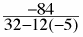3.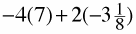4.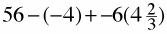Make sure you simplify each expression within parentheses first! Then you can multiply from left to right before finally subtracting.

−52.

Here, you should simplify the denominator (the bottom expression) before dividing.

Remember to multiply before subtracting! Also, don't forget that multiplying a negative integer and a positive integer is like removing groups of the same number.

Refer to the second hint in part (b) as well as the Math Notes box below for help simplifying this expression.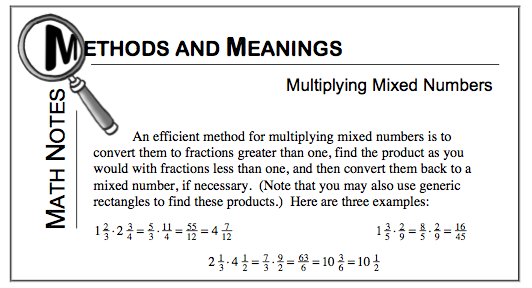Using the suggestions from parts (a), (b), and (c), along with the Math Notes box below, try your best!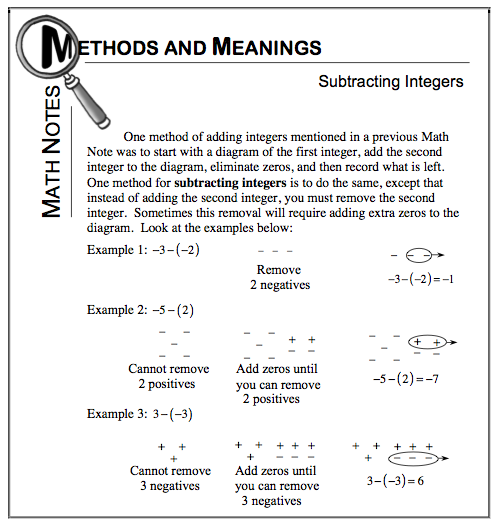Try circling the terms you can identify before simplifying. Also, showing all of your work can help you avoid unecessary mistakes!

The answer to part (d) is 32.